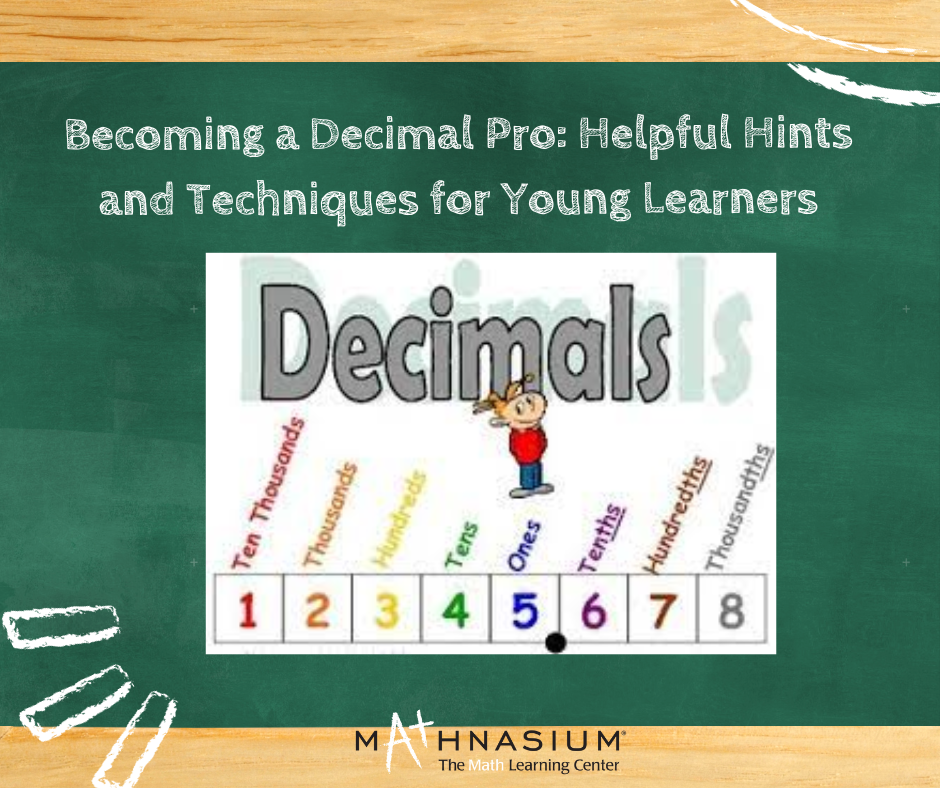877-601-6284
Get Started by Finding a Local Center

# Mastering Decimals: Tips and Tricks for Kids

Oct 3, 2023 | Point LomaDecimals can be tricky for kids to grasp, but with the right guidance and some fun techniques, they can become decimal masters in no time. In this blog, we'll explore some tips and tricks to help kids understand and master decimals.

1. Understanding the Basics: Before diving into decimals, it's essential to ensure that kids have a solid grasp of basic arithmetic, including addition, subtraction, multiplication, and division. These foundational skills will make it easier for them to work with decimals.

2. Visual Aids: Visual aids can be incredibly helpful when teaching decimals to kids. Use drawings, diagrams, and even physical objects like coins or fraction bars to illustrate decimal concepts. For example, show them that 0.5 is half of 1 by using a pie chart or drawing a pizza cut in half.

3. Relate to Real Life: Show kids how decimals are used in everyday life. Point out decimal numbers on price tags, thermometers, or measuring cups. This real-world context helps them understand the practical importance of decimals.

4. Decimal Place Value: Explaining the concept of place value is crucial. Teach kids that each digit in a decimal number has a specific value, such as tenths, hundredths, and thousandths. Use visual aids to represent these place values, making their grasp easier.

5. Decimal Conversion: Help kids understand the relationship between decimals, fractions, and percentages. For example, show them that 0.25 is the same as 1/4 and 25%. Use concrete examples to make these conversions more accessible.

6. Decimal Operations: Teach kids how to perform basic operations with decimals. Start with simple addition and subtraction, gradually moving on to multiplication and division. Encourage them to practice these operations using real-life problems.

7. Estimation: Teach kids to estimate decimal answers to problems before calculating them. This skill helps develop their number sense and ensures that their final answers make sense in the context of the problem.

8. Fun Games and Activities: Learning decimals can be enjoyable through games and activities. Use games like "Decimal Bingo" or "Decimal Jeopardy" to make learning engaging and interactive. You can also use online resources and apps that offer interactive decimal practice.

9. Fraction-Decimal Equivalents: Help kids understand that some fractions can be expressed as decimals, such as 1/2 being equal to 0.5. Show them how to convert these fractions to decimals and vice versa.

10. Real-Life Problem Solving: Present kids with real-life problems that require decimal calculations. For example, ask them to calculate the total cost of items with decimal prices or measure ingredients for a recipe using decimal measurements. These practical applications reinforce their decimal skills.

11. Practice, Practice, Practice: Encourage regular practice. Provide worksheets and exercises that gradually increase in complexity. Consistent practice helps reinforce what kids have learned and builds their confidence.

12. Patience and Positive Reinforcement: Be patient and provide positive reinforcement. Celebrate their successes, no matter how small, and offer support when they face challenges. A positive and encouraging environment fosters a love for learning.

13. Seek Help When Needed: If a child is struggling with decimals, don't hesitate to seek additional help. Teachers, tutors, and online resources can provide extra support and different teaching methods tailored to the child's needs.

In conclusion, mastering decimals doesn't have to be a daunting task for kids. With a combination of visual aids, real-world examples, interactive activities, and lots of practice, children can develop a strong grasp of decimals and build a solid foundation in mathematics. By making learning fun and relatable, you can help them become confident decimal masters. So, go ahead and explore these tips and tricks to unlock the world of decimals for your kids, helping them succeed in math and beyond.

Locations near

Could not find Center, try again